The Euler characteristic of an orbifold

Next: Positive and negative Up: Geometry and the Imagination Previous: The orbifold shop

# The Euler characteristic of an orbifold

Suppose we have a symmetric pattern in the plane. We can make a symmetric map by subdividing the quotient orbifold into polygons, and then `unrolling it' or `unfolding it' to get a map in the plane.

If we look at a large area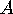in the plane, made up from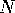copies of a fundamental domain, then each face in the map on the quotient orbifold contributesfaces to the region. An edge which is not on a mirror also contributes approximatelycopies - approximately, because when it is on the boundary of, we don't quite know how to match it with a fundametnal region.

In general, if an edge or point has order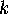symmetry which which preserves it, it contributes approximately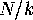copies of itself to, since each time it occurs, as long as it is not on the boundary of, it is counted incopies of the fundamental domain.

Thus,

• If an edge is on a mirror, it contributes only approximately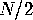copies.
• If a vertex is not on a mirror and not on a cone point, it contributes approximatelyvertices to.
• If a vertex is on a cone point of orderit contributes approximately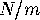vertices.
• If a vertex is on a mirror but not on a corner reflector, it contributes approximately.
• If a vertex is on an ordercorner reflector, it contributes approximately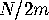Question. Can you justify the use of `approximately' in the list above? Take the area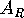to be the union of all vertices, edges, and faces that intersect a disk of radius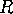in the plane, along with all edges of any face that intersects and all vertices of any edge that intersects. Can you show that the ratio of the true number to the estimated number is arbitrarily close to 1, forhigh enough?

Definition. The orbifold Euler characteristic is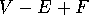, where each vertex and edge is given weight, whereis the order of symmetry which preserves it.

It is important to keep in mind the distinction between the topological Euler characteristic and the orbifold Euler characteristic. For instance, consider the billiard table orbifold, which is just a rectangle. In the orbifold Euler characteristic, the four corners each count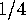, the four edges count, and the face counts 1, for a total of 0. In contrast, the topological Euler characteristic is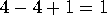.

Theorem. The quotient orbifold of for any symmetry pattern in the Euclidean plane which has a bounded fundamental region has orbifold Euler number 0.

Sketch of proof: take a large area in the plane that is topologically a disk. Its Euler characteristic is 1. This is approximately equal totimes the orbifold Euler characteristic, for some large, so the orbifold Euler characteristic must be 0.

How do the people at The Orbifold Shop figure its prices? The cost is based on the orbifold Euler characteristic: it coststo lower the orbifold Euler characteristic by 1. When they install a fancy new part, they calculate the difference between the new part and the part that was traded in.

For instance, to install a cone point, they remove an ordinary point. An ordinary point counts 1, while an ordercone point counts, so the difference is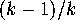.

To install a handle, they arrange a map on the original orbifold so that it has a square face. They remove the square, and identify opposite edges of it. This identifies all four vertices to a single vertex. The net effect is to remove 1 face, remove 2 edges (since 4 are reduced to 2), and to remove 3 vertices. The effect on the orbifold Euler characteristic is to subtract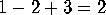, so the cost is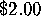.

Question. Check the validity of the costs charged by The Orbifold Shop for the other parts of an orbifold.

To complete the connection between orbifold Euler characteristic and symmetry patterns, we would have to verify that each of the possible configurations of parts with orbifold Euler characteristic 0 actually does come from a symmetry pattern in the plane. This can be done in a straightforward way by explicit constructions. It is illuminating to see a few representative examples, but it is not very illuminating to see the entire exercise unless you go through it yourself.

Next: Positive and negative Up: Geometry and the Imagination Previous: The orbifold shop

Peter Doyle